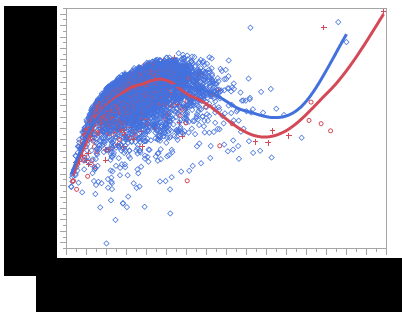Choose Language Hide Translation Bar
Highlighted

## Looping Bivariate Model

Hi,

I'm new to jmp script. But I know the gui interface. I would like to create multiple bivariate fits on the same graph using a loop? How will I do this? The "Fit Where" statement inside bivariate model doesn't work inside a loop. I want output like the following. There will be multiple fit spline curves, grouped by a column (:Experiment). I wish something like the commented section in the attached script would work. Any step by step guide will also help. I will figure out details of those steps to apply specifically to my data.

Thanks.``````dt = Current Data Table();

// Get the list of unique expts
Summarize( dt, ExptList = by( :Experiment ) );

Bivariate(
Y( :temp_Y ),
X( :temp_X ),

/*
For( i = 1, i <= N Items( ExptList ), i++,

Fit Where(
:Experiment == ExptList[i],
Fit Spline( 1, Standardized, {Line Color( {66, 112, 221} )} )
)

),
*/
SendToReport( Dispatch( {}, "Bivar Plot", FrameBox, {Marker Size( 1 )} ) )
);``````

2 ACCEPTED SOLUTIONS

Accepted Solutions
Highlighted

## Re: Looping Bivariate Model

All you need to do, is to use the GroupBy() setting

``````Names Default to Here( 1 );
dt = Current Data Table();

Bivariate(
Y( :temp_Y ),
X( :temp_X ),
group by( :Experiment ),
Fit Spline( 1000000 )
);``````
Jim
Highlighted

## Re: Looping Bivariate Model

You could also do it this way

``````dt = Current Data Table();

// Get the list of unique expts
Summarize( dt, ExptList = by( :Experiment ) );

biv = Bivariate(
Y( :height ),
X( :weight ),
SendToReport(
Dispatch(
{},
"Bivar Plot",
FrameBox,
{Marker Size( 1 )}
)
)
);

For( i = 1, i <= N Items( ExptList ), i++,

biv << Fit Where(
:Experiment == ExptList[i],
Fit Spline( 1 )
)

);	``````
Jim
3 REPLIES 3
Highlighted

## Re: Looping Bivariate Model

All you need to do, is to use the GroupBy() setting

``````Names Default to Here( 1 );
dt = Current Data Table();

Bivariate(
Y( :temp_Y ),
X( :temp_X ),
group by( :Experiment ),
Fit Spline( 1000000 )
);``````
Jim
Highlighted

## Re: Looping Bivariate Model

Hi Jim,
Thanks for the quick reply. Let me try this. I'll get back if I face any issue.
Highlighted

## Re: Looping Bivariate Model

You could also do it this way

``````dt = Current Data Table();

// Get the list of unique expts
Summarize( dt, ExptList = by( :Experiment ) );

biv = Bivariate(
Y( :height ),
X( :weight ),
SendToReport(
Dispatch(
{},
"Bivar Plot",
FrameBox,
{Marker Size( 1 )}
)
)
);

For( i = 1, i <= N Items( ExptList ), i++,

biv << Fit Where(
:Experiment == ExptList[i],
Fit Spline( 1 )
)

);	``````
Jim
Article Labels

There are no labels assigned to this post.